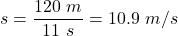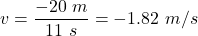## Anand gets into an elevator and travels 50 m straight up in a time of 5 s. He then travels in the opposite direction, straight down, for 70

Question

Anand gets into an elevator and travels 50 m straight up in a time of 5 s. He then travels in the opposite direction, straight down, for 70 m in a time of 6 s. What is his average speed and velocity, respectively, over the 11 s period?

A. 10.9 m/s, 1.82 m/s
B. 10.9 m/s, −1.82 m/s
C. 21.8 m/s, 10.9 m/s
D. −10.9 m/s, 5.2 m/s

in progress 0
2 weeks 2021-09-05T16:44:08+00:00 1 Answers 0 views 0

10.9 m/s, −1.82 m/s

Explanation:

We have,

Anand gets into an elevator and travels 50 m straight up in a time of 5 s. He then travels in the opposite direction, straight down, for 70 m in a time of 6 s.

Total time of journey is 11 seconds.

Total distance is 50 m + 70 m = 120 m

Total displacement is 50 m – 70 m = -20 m

Average speed,Average velocity,So, the correct option is (b) 10.9 m/s, −1.82 m/s.